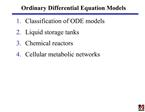DownloadDownload PresentationOrdinary Differential Equation Models

# Ordinary Differential Equation Models

Download Presentation## Ordinary Differential Equation Models

- - - - - - - - - - - - - - - - - - - - - - - - - - - E N D - - - - - - - - - - - - - - - - - - - - - - - - - - -
##### Presentation Transcript

1. Ordinary Differential Equation Models Classification of ODE models Liquid storage tanks Chemical reactors Cellular metabolic networks

2. Mathematical Modeling Motivation Yields improved understanding of physicochemistry Provides rigorous framework for data analysis Allows prediction without additional experimentation Core competency of any chemical engineer UMass ChE curriculum ChE 120 � mass and energy balance modeling ChE 226, 325 � thermodynamic modeling ChE 230 � fluid transport modeling ChE 320 � chemical reaction modeling ChE 333 � heat and mass transfer modeling ChE 338 � separation unit modeling ChE 444 � plant-wide modeling ChE 446 � control system modeling

3. Classification of ODE Models Order Linearity Explicit versus implicit

4. Classification of ODE Models cont. Dimension Current focus: systems of first-order, explicit ODEs

5. Liquid Storage Tank Standing assumptions Constant liquid density r Constant cross-sectional area A Other possible assumptions Steady-state operation Outlet flow rate w0 known function of liquid level h

6. Mass Balance Models Mass balance on tank Steady-state operation: Valve characteristics Linear ODE model Nonlinear ODE model

7. Stirred Tank Chemical Reactor Assumptions Pure reactant A in feed stream Perfect mixing Constant liquid volume Constant physical properties (r, k) Isothermal operation

8. Plug-Flow Chemical Reactor Assumptions Pure reactant A in feed stream Perfect plug-flow Steady-state operation Isothermal operation Constant physical properties (r, k)

9. Plug-Flow Chemical Reactor cont. Overall mass balance

10. Cellular Metabolic Networks

11. Yeast Glycolysis

12. Model Formulation Intracellular concentrations Intermediates: S1, S2, S3, S4 Reducing capacity (NADH): N2 Energy capacity (ATP): A3 Reaction scheme

13. Stoichiometric Model Assumptions Intracellular and extracellular steady state Measure glucose influx J0 and acetaldehyde/pyruvate efflux J Steady-state mass balances

14. Kinetic Model Reaction scheme Reaction kinetics for v2-v6 Reaction kinetics for v1

15. Kinetic Model cont. Intracellular mass balances Conserved quantities ODE model